## 1. 循环神经网络 RNN

• 张三非常生气，冲动之下打了李四
• 李四非常生气，冲动之下打了张三

### 1.1. 循环神经网络RNN是什么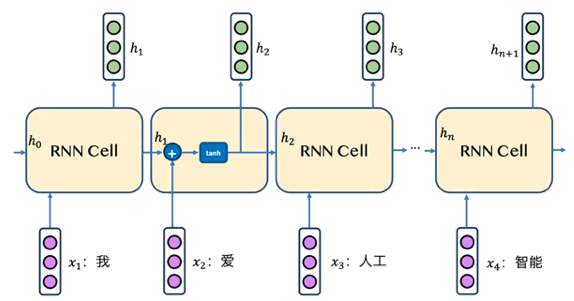Tip：当RNN读完最后一个单词后，那RNN就已经读完了整个句子，一般可认为最后一个单词输出的状态向量能够表示整个句子的语义信息，即它是整个句子的语义向量，这是一个常用的想法。

### 1.2. RNN的公式推导

$$h_t = tanh(Wx_t + Vh_{t-1}+b)$$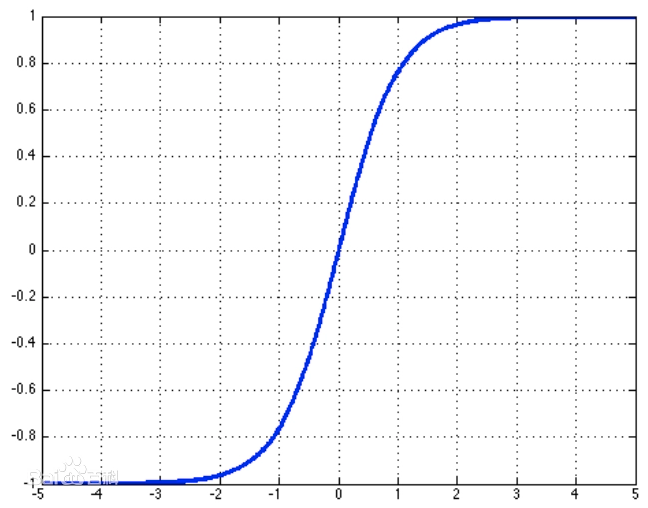### 1.4. RNN的几种常见模式

• 序列到类别模式
• 同步的序列到序列模式
• 异步的序列到序列模式

#### 1.4.1. 序列到类别模式

1.1. 中讲到，在RNN读完一个句子的最后一个单词后，该单词对应的输出便可以看做能够代表整个句子的语义向量，根据这个语义向量可以进一步计算一些任务，比如文本分类，假设通过这个语义向量能够将"我爱人工智能"这句话划分为"科技"类别，那这就是一个完整的序列到类别模式的应用。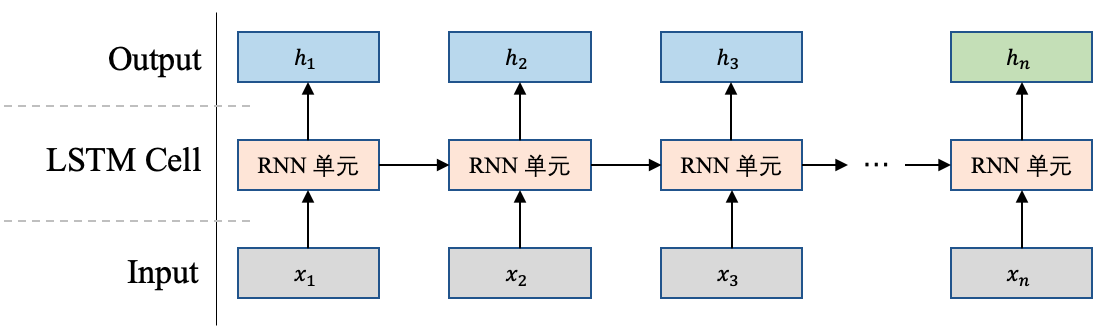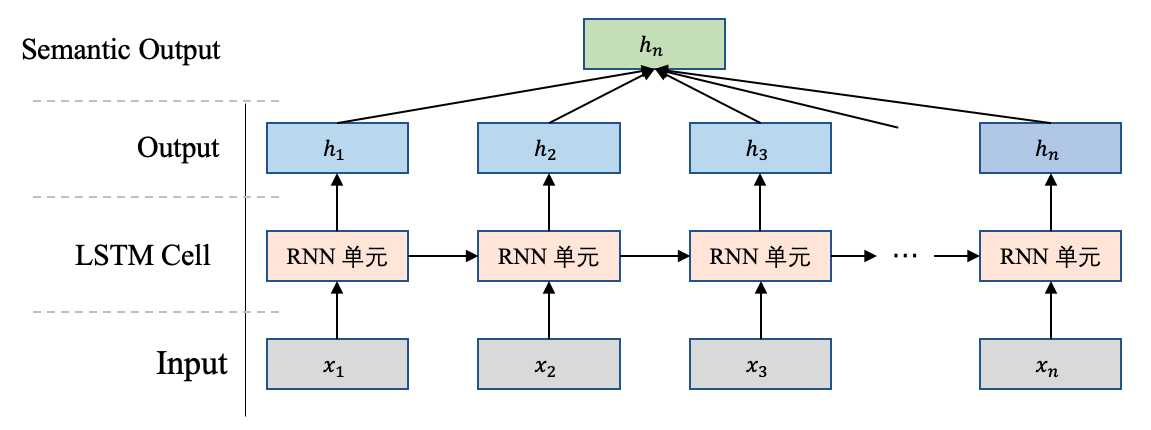#### 1.4.2. 同步的序列到序列模式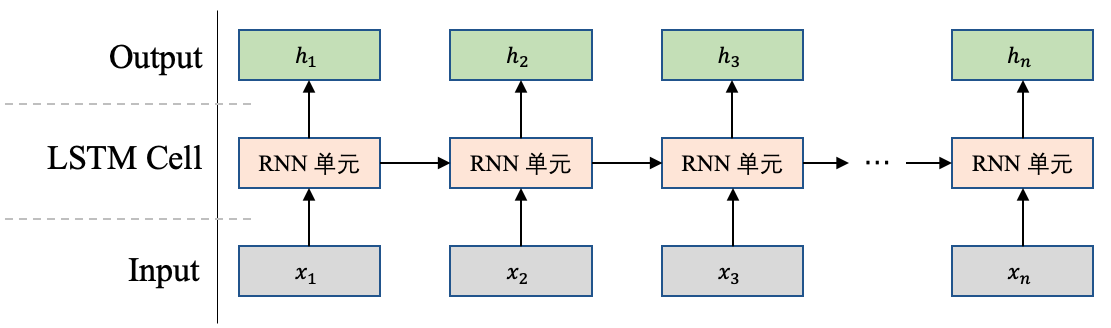#### 1.4.3. 异步的序列到序列模式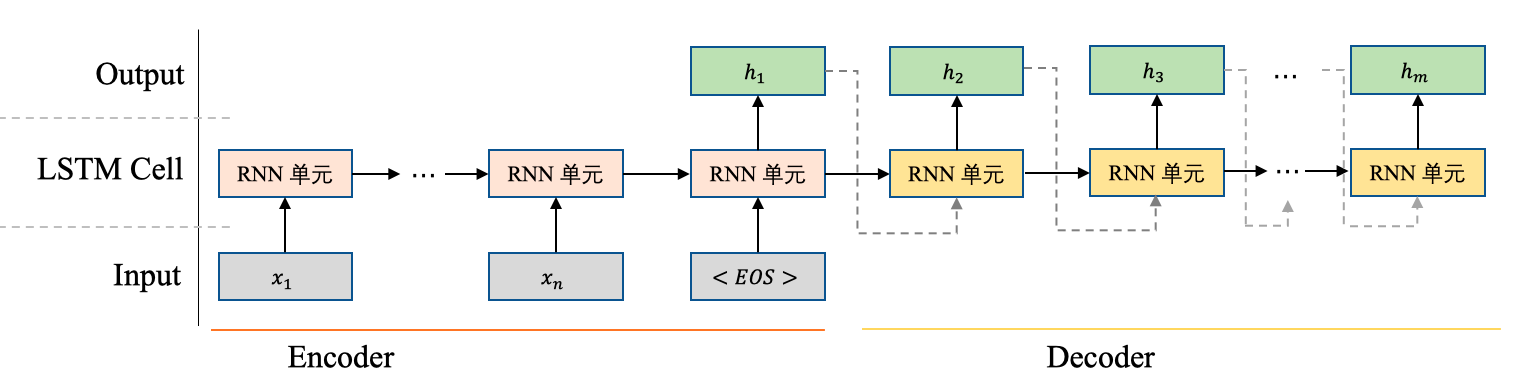## 2. 长短时记忆网络 LSTM

### 2.2. LSTM是怎样工作的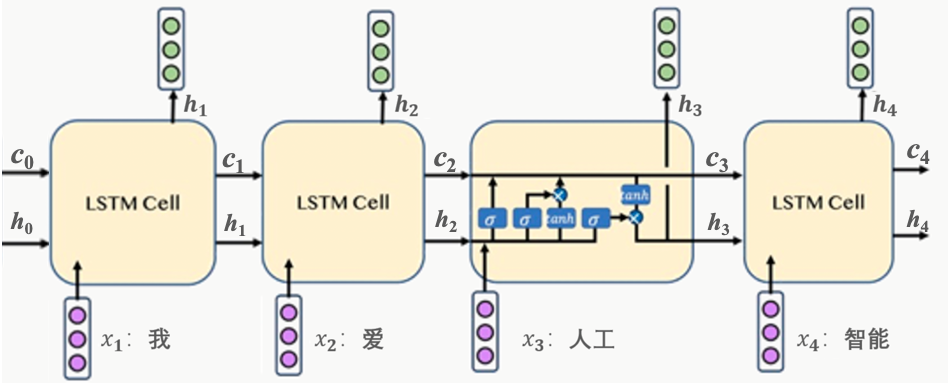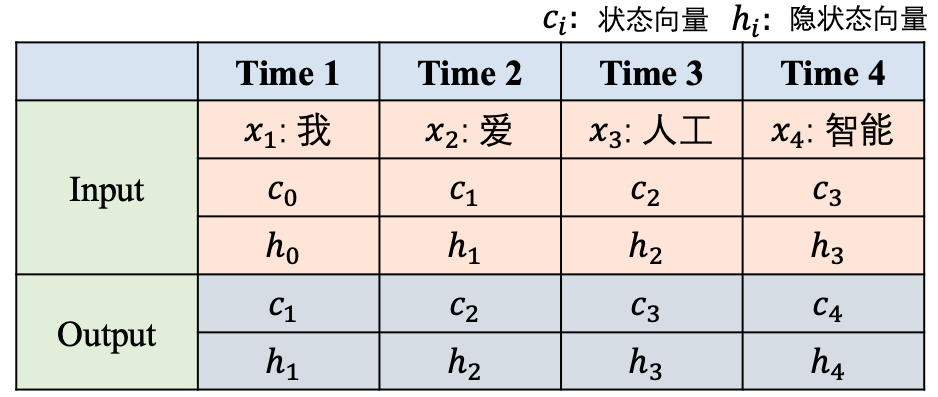### 2.3. 从公式层面理解LSTM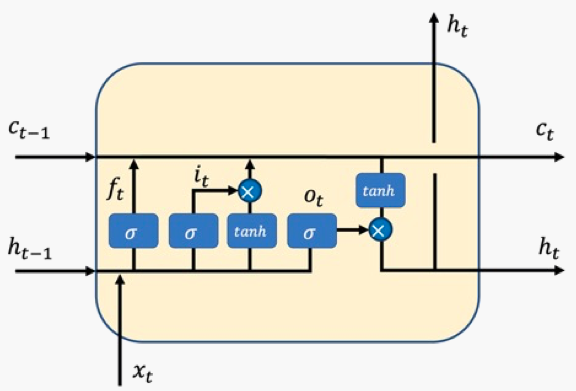• 状态向量$c_t$：它控制着整个LSTM单元的状态或者记忆，它会根据每个时刻的输入进行更新，从而实时保持LSTM单元的记忆。
• 隐状态向量$h_t$：它是当前LSTM单元对外的输出状态，它是实际的工作状态向量，即一般会利用$h_{t}$来做一些具体的任务。
• 输入门$i_t$：控制当前时刻的输入信息需要向状态向量$c_t$中注入哪些信息。举个例子，当输入信息是一些没有实际意义的词，比如"的"，可能模型不会让这些信息流入到状态向量中，从而保持模型的语义表达。
• 遗忘门$f_t$：控制前一时刻的状态向量$c_{t-1}$需要被屏蔽/遗忘哪些信息。举个例子，昨天我去爬了长城，哦不对是前天， 当模型看到"不对，是前天"的时候，可能就会忘记前边的"昨天"。
• 输出门$o_t$：控制当前时刻的状态向量$c_t$需要对外输出哪些信息，最终输出的信息即为$h_t$。

\begin{align} i_t &= sigmoid(W_ix_t+U_ih_{t-1}+b_i) \\ f_t &= sigmoid(W_fx_t+U_fh_{t-1}+b_f) \\ o_t &= sigmoid(W_ox_t+U_oh_{t-1}+b_o) \end{align}

$$a_t = tanh(W_ax_t+U_ah_{t-1}+b_a)$$

$$c_t = f_t \cdot c_{t-1} + i_t \cdot a_t$$

$$h_t=o_t \cdot tanh(c_t)$$

### 2.4. 使用LSTM设计情感分析任务

#### 2.4.1. 情感分析是什么#### 2.4.2. 使用LSTM进行文本分类建模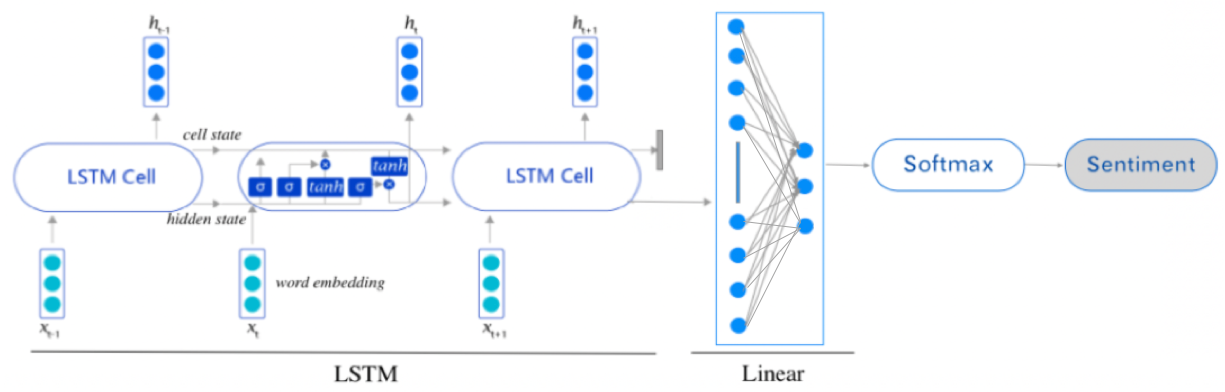## 3. 门控循环单元 GRU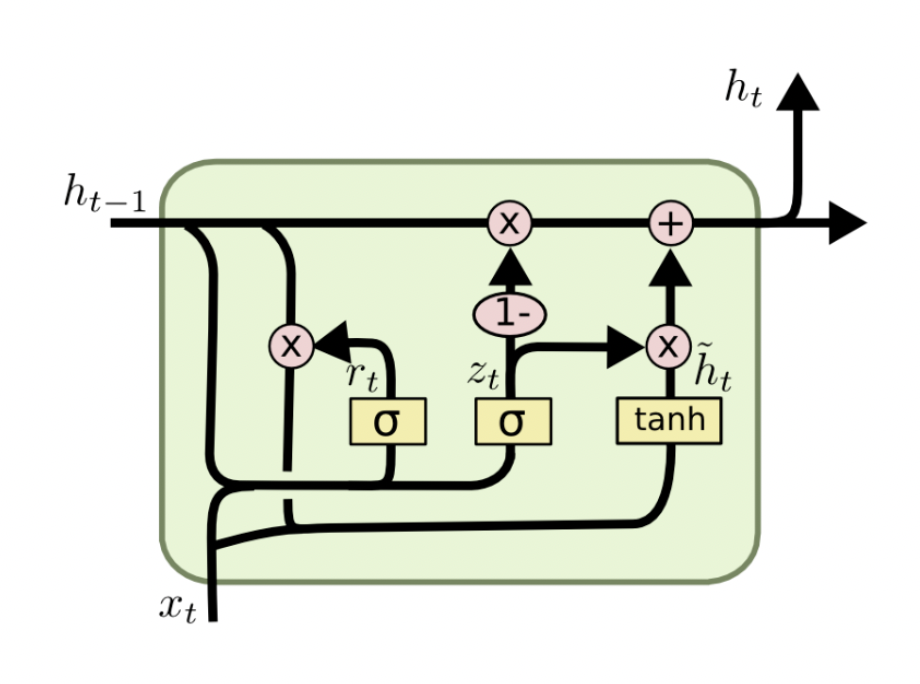GRU在LSTM的基础上主要做出了两点改变 ：

 - GRU只有两个门。GRU将LSTM中的输入门和遗忘门合二为一，称为更新门（update gate），上图中的$z_{t}$，控制前边记忆信息能够继续保留到当前时刻的数据量，或者说决定有多少前一时间步的信息和当前时间步的信息要被继续传递到未来；GRU的另一个门称为重置门（reset gate），上图中的$r_{t}$ ，控制要遗忘多少过去的信息。

 - 取消进行线性自更新的记忆单元（memory cell），而是直接在隐藏单元中利用门控直接进行线性自更新。GRU的逻辑图如上图所示。

GRU的公式化表达如下：

$$z_{t}=\sigma(W_{z} \cdot [h_{t-1},x_{t}])$$
$$r_{t}=\sigma(W_{r} \cdot [h_{t-1},x_{t}])$$
$$\tilde h=tanh(W \cdot [r_{t} \odot h_{t-1},x_{t}])$$
$$h_{t}=(1-z_{t})\odot h_{t-1}+z_{t} \odot \tilde h_{t-1}$$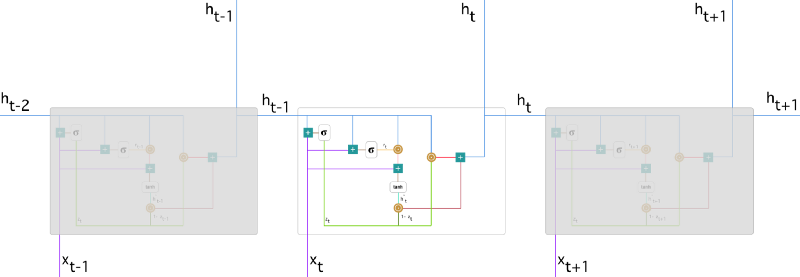### 3.1. 更新门

$$z_{t}=\sigma(W_{z} \cdot [h_{t-1},x_{t}])$$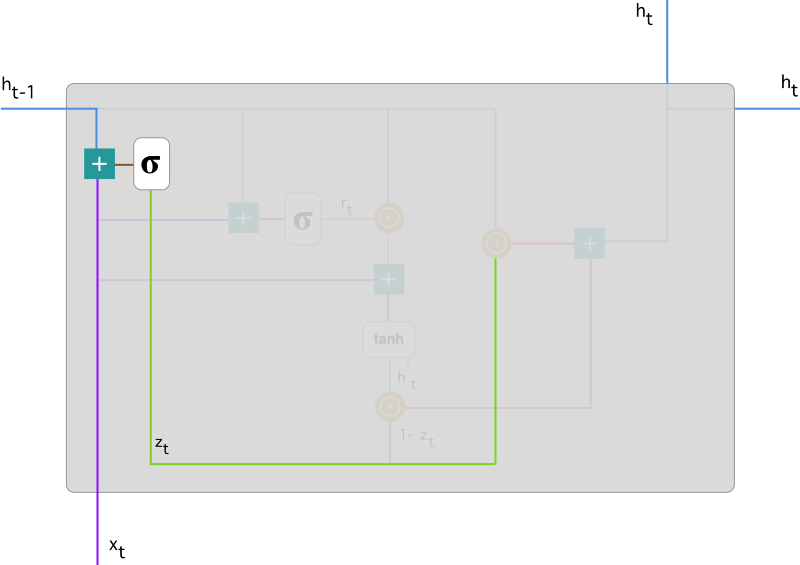### 3.2. 重置门

$$r_{t}=\sigma(W_{r} \cdot [h_{t-1},x_{t}])$$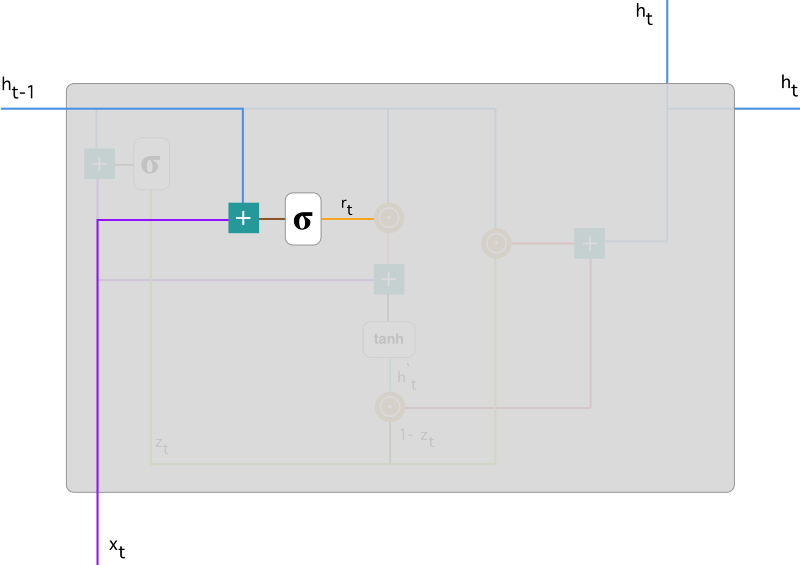### 3.3. 当前记忆内容

$$\tilde h=tanh(W \cdot [r_{t} \odot h_{t-1},x_{t}])$$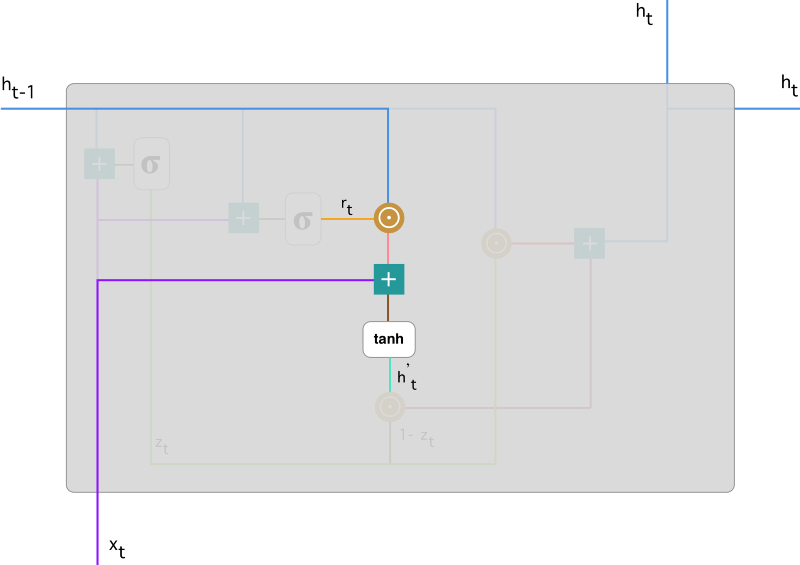### 3.4. 当前时间步的最终记忆

$$h_{t}=(1-z_{t})\odot h_{t-1}+z_{t} \odot \tilde h_{t-1}$$

$z_t$ 为更新门的激活结果，它同样以门控的形式控制了信息的流入。$1-z_t$ 与$h_{t-1}$的 Hadamard 乘积表示前一时间步保留到最终记忆的信息，该信息加上当前记忆保留至最终记忆的信息($z_t$ 与$\tilde h_{t-1}$的 Hadamard 乘积)就等于最终门控循环单元输出的内容。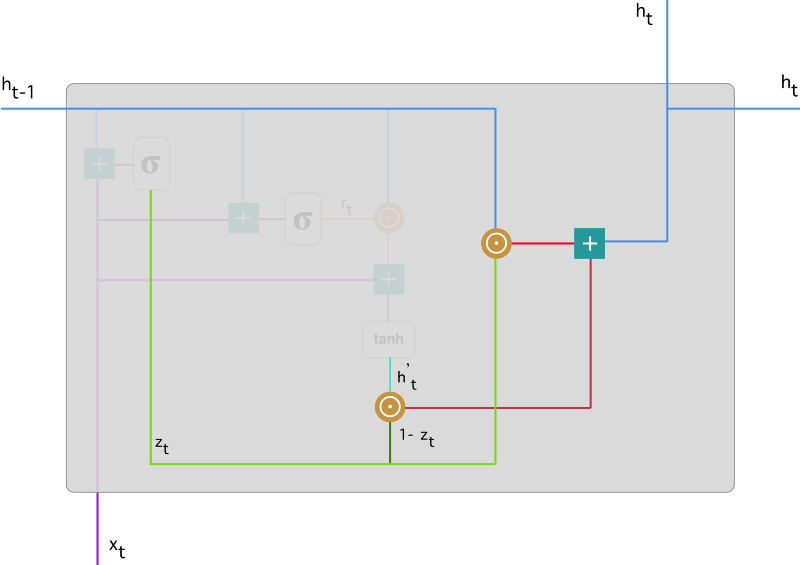### 参考文献

 - Chung, J., Gulcehre, C., Cho, K., & Bengio, Y. (2014). Empirical evaluation of gated recurrent neural networks on sequence modeling

Last modification：September 18th, 2021 at 11:21 pm